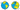# Latitude and Longitude To DMS Converter

Latitude in Decimal

Longitude in Decimal

Latitude in DMS

Longitude in DMS### DMS

Degrees minutes seconds (DMS) are a special type of units used for measuring angles, as an alternative to decimal way to stating the size of an angle.
It is a recognized fact of the full circle have 360 degrees, with 1/60th of those being 1 minute, and 1/60th of one minute being 1 second.
The numerical values for latitude and longitude in decimal number format:
degrees (°), for minutes ('), for seconds ('').
The common abbreviations of the directions:
S = South
W = West
E = East
N = North